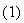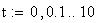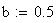Dynamic response from DPZTFFC device
by Navier-Stokes equation exact solutionBoundary layer theory was first developed by Prandtl. He showed that for a moving fluid all friction losses occur within a thin layer adjacent to a solid boundary (called the boundary layer) and that flow outside this layer can be considered frictionless. The velocity near the boundary is affected by boundary shear. In general, the boundary layer is very thin at the upstream boundaries of an immersed object but increases in thickness due to the continual action of shear stress.
At low Reynolds numbers, the entire boundary layer is governed by viscous forces, and laminar flow occurs therein. For high Reynolds numbers, the entire boundary layer is turbulent.
Flow described by Navier-Stokes equations. In general the problem of finding exact solutions of the NS equations presents insurmountable mathematical difficulties. This is primarily a consequence of their being non-linear.
Nevertheless, it is possible to find exact solutions in certain particular cases.

In case of small viscosity many of the exact solutions have a boundary-layer structure which means that the influence of viscosity is confined to thin layer near the wall.
It can be seen that the thickness of the boundary layer (d ) will increase as the square root of character dimension x increases and also as the square root of the kinematic viscosity increases, while d will decrease as the square root of the velocity increases. Similarly, the boundary shear t0 will increase as r and m increase, will decrease as the square root of x increases, and will increase as the square of V increases.
Let's discuss the parallel flows, constitute a particularly simple class of motions. A flow is called parallel if only one velocity component is a different from zero and all fluid particles are moving in the one direction.
For irrotational, incompressible flow with F=0 , the Navier-Stokes equation then simplifies toFor low Reynolds number, t he inertia term is smaller than the viscous term and can therefore be ignored, leaving the equation of creeping motion:In creeping motion regime, viscous interactions have an influence over large distances from an obstacle.
For low Reynolds number, flow at low pressure, the Navier-Stokes equation becomes a diffusion equation:This type of flow would be prevail flow inside channels of DPZTFFC device and we must discuss it first.
Equation (3) with the boundary conditions u=0 for y=+/-b describe steady flow in channel between two parallel plates.
And solution for this case is parabola.

Another simple solution of equation (3) is obtained for so-called Couette flow between two parallel flat walls, one of which is a rest the another moving in its own plane with constant velocity.

We will investigate another application for equation (3) - the flow near oscillating flat plate - the Stokes's second problem.
Case 1.
Consider the laminar flow of incompressible liquid near an oscillatory plate.This is a speed of plate oscillation (at the wall /plate y=0), with U - amplitude of speed and w - frequency.
The second B.C. is u(oo,t)=0The solution is:whereand- is kinematic viscosity
Let's compute this equation for two different frequencies 5 and 50 rad/sec:The velocity profile has the form of a damped harmonic oscillation in which a fluid layer at a distance y has a phase lag
ky with respect to the motion of the wall.
The layer which is a carried by the wall has a thickness proportional to: and decreases for decreasing kinematic viscosity and increasing frequency.Case 2
Consider now more complicate problem: a plane laminar flow of incompressible viscous fluid between two parallel plates separated by distance 2*b. The bottom plate moves, oscillates with velocity u(0,t)=Ucos(w.t) , while the upper plate is held stationary, so that B.C. is u(2b,t)=0 . Initial condition: u(y,0)=Uo*(1-y/2b).Will solve the same diffusion equation (3) with different B.C. and I.C.
In this case the solution is:This is an attempt to solve same problem,
same case but different way.
Developed by Roman N Tunkel,
Research Engineer, Ph.D.,
tunkelrn@utrc.utc.com